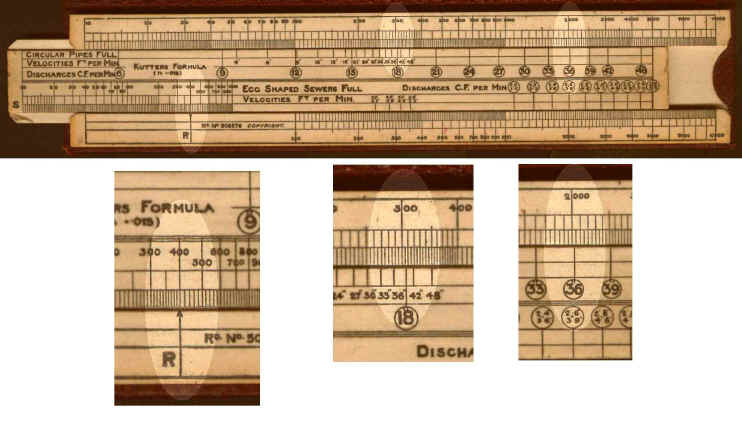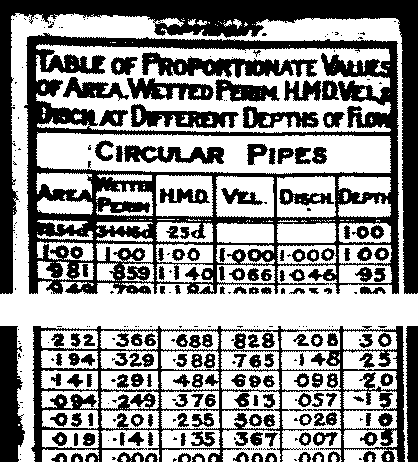Instructions for using the EASYR Hydraulic Slide Rule

Background

One of the fundamentals of sewerage design is choosing the gradient and shape of the sewer so that no settlement of solid material occurs at low flows but so that maximum capacity is obtained at high flows. This was a particular problem in early sewerage systems, known as combined systems, with foul (domestic) effluent flowing in the same pipe as surface runoff from rainfall. The range of discharges was therefore very high.

Whilst many sewers are circular in cross-section the use of egg shaped sewers was found to be good compromise designed to achieve the required flow velocities and capacity. The calculation of the velocities and discharges for different gradients and sizes of sewers was a complex, but everyday, problem faced in sewer design. A further complication  was the need to calculate these values when the pipe was not running full. The EASYR (easier - get it) rule was designed tackle this problem.

The rule

The rule allowed the problem to be solved in two stages.

In the first stage the rule was used to get the velocity and discharge for a given gradient and sewer dimension when the pipe is running full. At the bottom end the values were for egg shaped sewers and at the top end for circular sewers. The calculations were performed using the Kutter formula, on one face of the slide, and using the Crimp and Bruges formula, on the reverse face.

For the second stage there was a table of values of proportional discharge and velocity for pipes which are partially full.

Example

Using the Kutter formula, what are the velocity and flow for a pipe 36" diameter and a gradient of 1 in 400 which is flowing at 10% of its maximum depth.Set the R against the gradient (1 in 400). Read the velocity 288 ft/min against the small 36" sign. Read the discharge 2020 ft3/min against the larger 36" sign (enclosed in the circle).From the table attached to the rule, against 0.1 proportional depth read 0.506 as proportional velocity and 0.026 as proportional discharge. This gives a velocity of 146 ft/min (288 x 0.506) and a discharge of 52.5 ft3/min.